The bonds which are issued by U.S. Government are called Treasury bonds. This is no uncertainty when it comes to repayment of treasuries. The debt which is associated with treasury is issued by the treasury department of the U.S. Treasuries are of three types:

1. Treasury Bills or T-Bills which have maturity of less than a year.
2. Treasury notes whose maturity can range from 1 to 10 years.
3. Treasury bonds which have maturity of more than 10 years.

Those bonds which have a period of 30 years are called long bonds.

In the month of October 2004, the U.S. owned treasuries which were worth \$7.4 trillion. Buyers and sellers of treasuries’ are found very easily therefore the transaction of treasuries do not pose any problem. Trading volume plays a very crucial role in the economy’s GDP. In 2004, the trading value was \$130 trillion per annum, which was nearly ten times of the economy’s GDP.

The interest rate on treasuries vary according to their terms of maturity. Yield curve plays an important role in determining the interest rate on treasuries. It is represented in the form of a graph, where the time of maturity is plotted on the x-axis while the annualized interest rates are plotted on the y-axis

REVIEW QUESTION

1.Explain the different types of treasuries.

5.3 A SHAPES OF YIELD CURVES

Generally, four shapes are taken into consideration while studying yield-curves. These are explained below:

1. Flat: When the difference between annualized short term and long term rates are very little, it gives rise to a flat curve.
2. Upward sloping: upward sloping curves are normal curves. Here, the short term rates are lower than long term rates.
3. Downward sloping: The short term rates are higher than long term rates. This is the inverted curve.
4. Humped: Long and short-term rates are lower than medium rates.

These curves are illustrated below: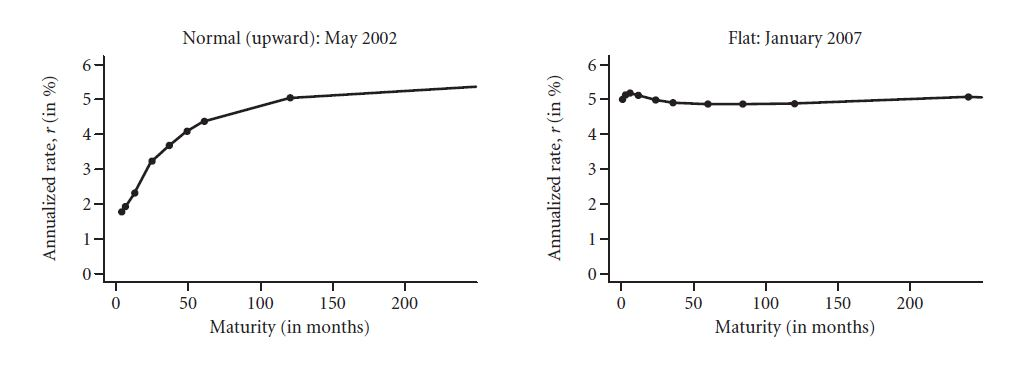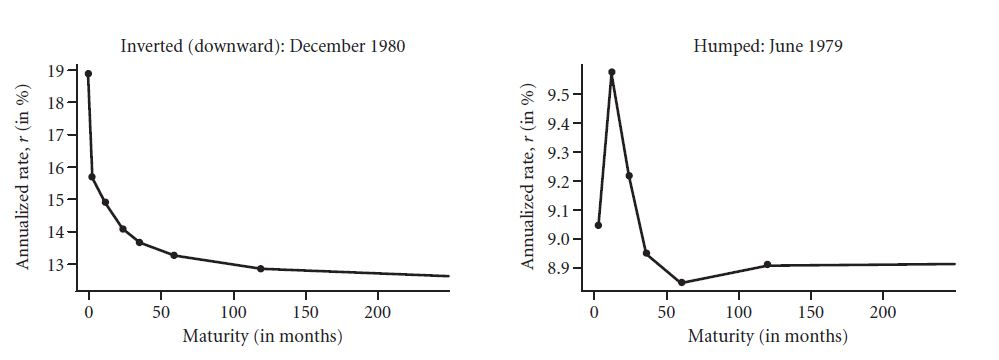5.3 B AN EXAMPLE: THE YIELD CURVE OF DECEMBER 31, 2004

The situation of December 31, 2004 has been explained with the help of a graph. As we can see, it is an upward-slopping graph. It says that a three month treasury bought would have yielded an annual interest rate of 1.63% per annum and a purchase of a 20-year bond would have yielded an annual interest rate of 4.85% per annum.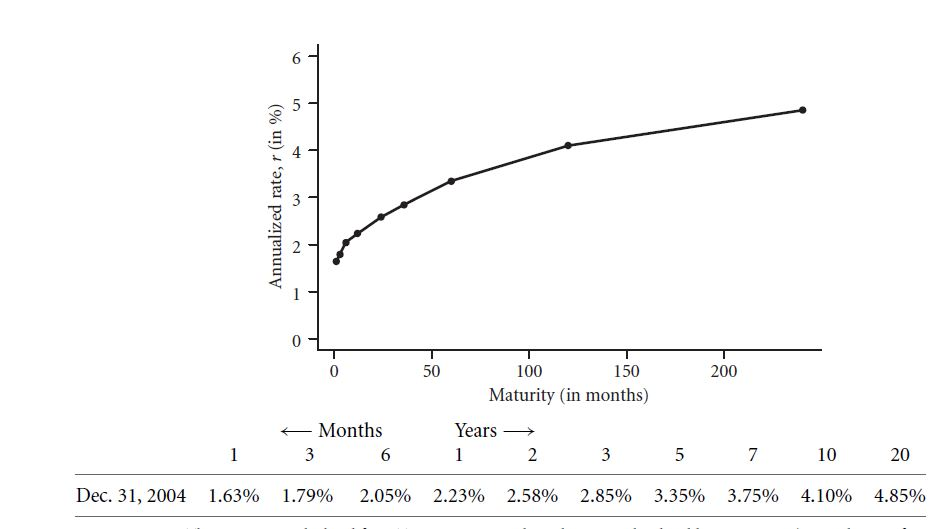The process of interpolation is used for determining an interest rate on a non-listed bond.

Forward rate of return:When investors buy a stock today, they expect a return value in future. This is known as forward rate of return.

Holding rate of return:The return which is realized by the investor during a real or expected value of time is called holding rate of return. It is calculated as the income plus the price appreciation during the specific year, divided by the cost of the investment.

5.3 C BOND PAYOFFS AND INVESTMENT HORIZON

There is no relation between the kind of bonds and your investment horizon. Let a 3-year bond offer 2.85% and the 1-year bond offer 2. 23%. If you want the money in one year, how will you do it? There is a provision of buying a 3-year bond today and selling it out the next year. At that time, it would have become a 2-year bond.

But, this investment strategy will give you only 2.23%, which the 1-year bond was providing you.

5.3 D EFFECT OF INTEREST RATE ON SHORT AND LONG TERM BONDS

It has often been found that long term bonds are riskier than short-term bonds. There is no uncertainty associated with payment of treasury bonds. Let us study the effect of sudden interest rate change on the maturity of the bonds. Let us understand this by studying a 20-year bond and a 1-year note.

If the interest rate is 4.85%, then a \$1000 20-year zero-bond investment will cost around \$387. If the interest rate will go up, you will be at a loss, and vice-versa. The effect of a 10 basis point will change the value of the bond in the following way: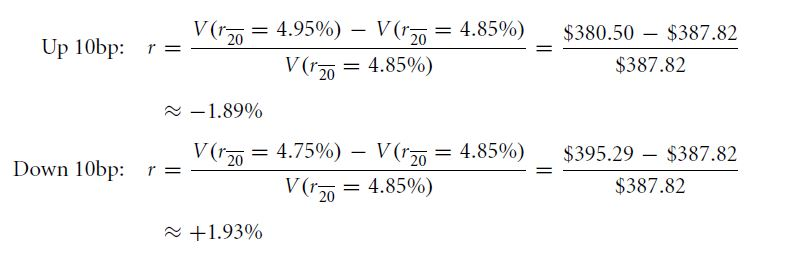Every \$1 million investment will result in a risk of \$19,000 in case of 20-year bond.

Let us now assume a bank note, which has the interest rate of 4.85 and a 10 basis point change, as the previous case. Here, the values of the computation are \$954.65 at 4.75%, \$953.74 at 4.85%, and \$952.83 at 4.95%. The instant rates of return are given by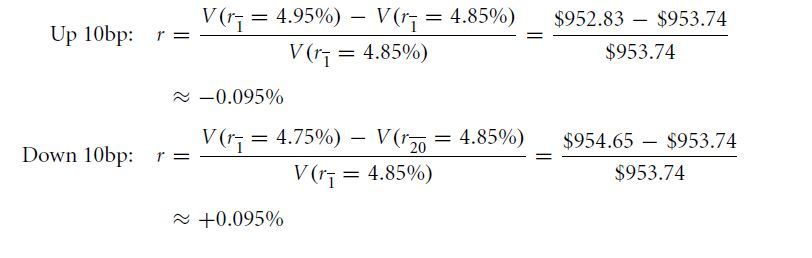Every \$1 million investment will result in a risk of \$9, 50 in case of the 1-year bank note.

The effect of rate of interest is more pronounced in long term bonds. Treasury bonds are said to be risk-free because they return the promised payment, but the interim interest rate can change their value. Therefore, in true sense only short-term Treasury Bills can be considered risk-free.

REVIEW QUESTIONS

1. A one-year and a 10-year zero bond, both offering an interest rate of 8% per annum.
2. What is the effect of an increase of 1 basis interest rate in the 1-year bond?
3. What is the effect of an increase of 1 basis interest rate in the 10-year bond?
4. Calculate the ratio of the changed values obtained in the above two questions. This is called the derivative of the value with respect to the change in the interest rate. Which one is the larger derivative?

Links of Next Financial Accounting Topics:-### Customer Reviews

My Homework Help
Rated 5.0 out of 5 based on 510 customer reviews at